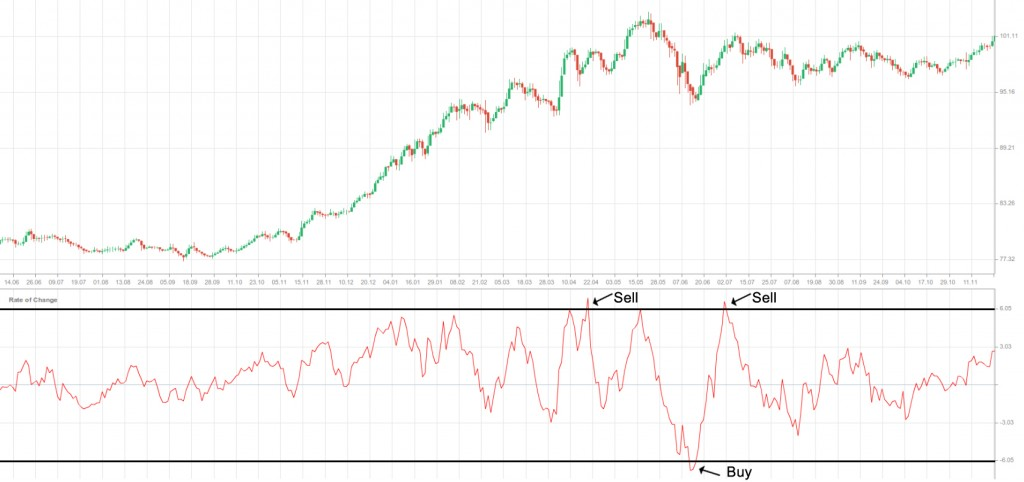# Rate of Change

## Rate of Change

### This lesson will cover the following

• What is Rate of Change?
• How is the ROC calculated?
• How can it be used in order to trade?

## Explanation and calculation

The Rate of Change (ROC) is a momentum oscillator, which measures the amount a price of a tradable instrument has changed during a specific number of past periods. It compares the current price with the price ”N” periods ago. As an oscillator, the ROC fluctuates above and below its zero value (zero line). It is usually calculated with the use of the following formula:

ROC = {(P today – P n periods ago)/ P n periods ago} x 100

## Best Forex Brokers for United States

The ROC will be equal to zero, if the current day’s price is the same as it was ”n” periods ago. It demonstrates how the current price relates to the past price on a continuous basis. Usually, prices are rising as long as the Rate of Change remains positive. Respectively, prices are declining as long as the Rate of Change remains negative. The oscillator can expand into positive territory, in case price advance accelerates. Conversely, the ROC can plunge deeper into negative territory, in case price decline accelerates.

If the ROC is above its zero line but decreasing, this indicates prices are still rising but at a slower pace. If the ROC is below its zero line but rising, this indicates the market is not declining as fast and the downtrend may be nearing its exhaustion.

## How can it be used in trading?As any other oscillator, the Rate of Change can be used in four major ways by traders. First, the ROCs position relative to the zero line can indicate the underlying trend. Second, it can be a divergence oscillator, showing when the momentum relative to the past is changing. Third, the ROC can produce identifiable extremes, which signal overbought or oversold conditions. And fourth, it can generate a signal when it crosses over the zero line. However, in none of the above mentioned instances is the signal reliable enough to be traded solely, without the help of other technical tools.

### Trend identification

Despite that momentum oscillators are used best during trading ranges or flat trends, they can also be used to identify the overall direction of the underlying trend. Within a year there are approximately 250 trading days and respectively, 125 trading days per half year, 63 trading days per quarter and 21 trading days per month. As a rule, the long-term trend is an uptrend, in case both the 250-day and 125-day Rate of Change are on positive territory. This means that current prices are higher than they were 12 and 6 months ago. Conversely, the long-term trend is a downtrend, in case both the 250-day and 125-day ROC are negative.

On this 1-hour chart of USD/JPY we can see that since January 23rd the 250-period ROC (the red oscillating line), the 125-period ROC (the green oscillating line) have moved below their zero line, indicating a strong downward price move. A few hours later the 63-period ROC (the blue oscillating line) has performed the same, which can be considered as a confirmation of the downtrend.

### Overbought and oversold signals

Some analysts consider that the most popular time periods are the 12-period and 25-period ROC, if we are engaged into short-term or intermediate-term trading. The 12-period Rate of Change makes a good short- to medium-term overbought/oversold indicator.

The higher the ROC is, the more overbought the instrument we trade is, and respectively, the greater the chance for a drop is. The lower the ROC is, the more oversold the tradable instrument is, and respectively, the greater the chance for a surge is. However, beginner traders should note that a market, which appears overbought, may remain overbought for some time. It is so, because extreme overbought and oversold readings usually suggest that the current trend may probably continue to develop.We placed “buy” signals each time the ROC fell below, and then rose above the oversold level of -6.0. We placed “sell” signals each time the ROC rose above, and then fell below the overbought level of +6.0. It again comes to experimentation when establishing the two extreme levels and, as we said before, the signals the ROC provides are not 100% reliable.

## Certain drawbacks

The ROC has certain drawbacks as an indicator. Although economists often calculate it with the use of macroeconomic data, usually on an annual basis in order to dampen seasonal effects, it suffers from the so called ”drop-off effect”. Only two prices, P today and P n periods ago, are presented in the calculation of the ROC, while these two prices are given an equal weighting. The older price, which occurred N periods ago, has the same effect as the current and probably more relevant price for the oscillator itself. The ROC can show a current increase or decrease based solely on what number drops off in the past. Some analysts may smooth the Rate of Change with a moving average in order to minimize this effect.